# OECD Road accidents data analysis 2 - R summary() function is very useful to see a data frame statistics.Photo by Clyde RS on Unsplash

This post is following of above post.
In the previous post, I made a data frame named "df".
Let's see the data frame in detail. I will use summary() function in R.

Before using summary() function, I convert iso to factor class.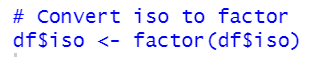I know there are three kinds of values in "subject", ACCI, DEATH and INJURE.
Let's see each subject data.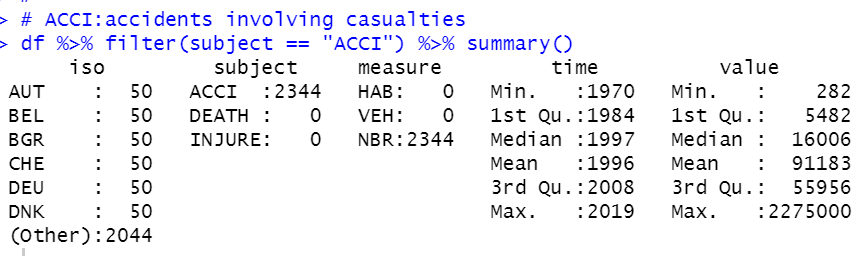ACCI is accidents involving casualties. We see ACCI has only NBR measure. NBR is number. We see time is from 1970 to 2019, it is 50 years. minimum value is 282 and max value is 2,275,000.

Then, let's see DEATH, it is death of course.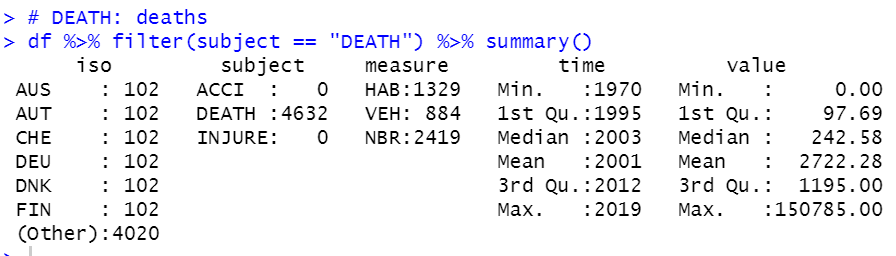We see DEATH has all three measure, HAB, VEH and NBR. So, let's see eaches.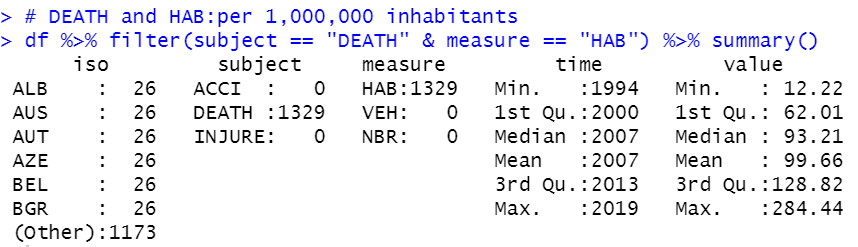DEATH & HAB: per 1,000,000 inhabitants has 1329 observations. time is from 1994 to 2019, 26 years data. the minimum value is 12.22 and the maxmum value is 284.44.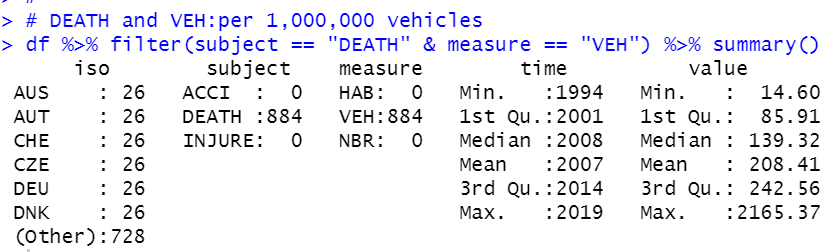DEATH and VEH: per 1,000,000 vehicles has 884 observations. time is from 1994 to 2019, 26 years. It is the same as DEATH and HAB. the minimum value is 14.60 and the maximum value is 2165.37. VEH has more variance than HAB.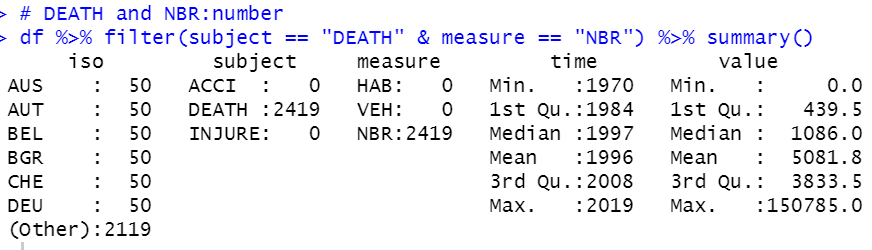DEATH and NBR: number has 2419 obserbations. time is from 1970 to 2019, 50 years.
The minimum value is 0 and the maximum value is 150,785.

All right, let's see INJURE: injuries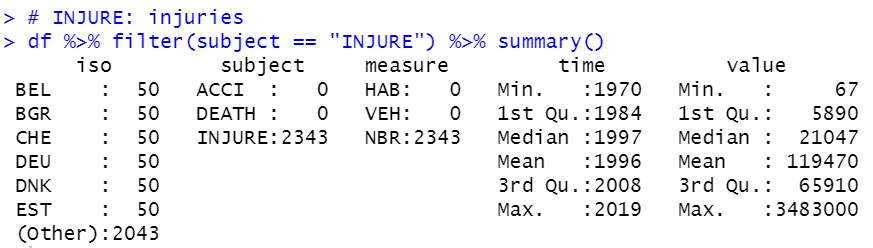INJURE has only one measure, NBR. The number of observations is 2343. time is from 1970 to 2019, 50 years. the minimum value is 67 and the maximum value is 3,483,000.

That's it. Thank you!

The next post is ..

To see the 1 st post